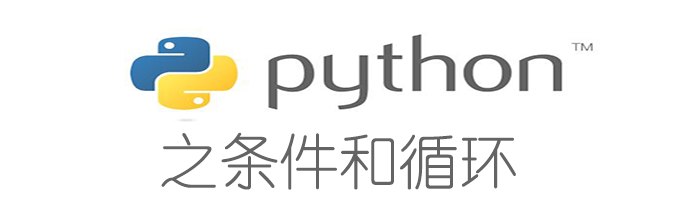# Python中的分支语句和循环语句及案例### if条件控制语句

``````money = 17
if money > 18:
print("今天吃鸡")
if 10 < money <= 18:
print("今天吃鱼")
if money == 0:
print("今天不吃了")``````

### if,elif,else条件判断语句

if else 条件，表示如果..否则..，if后面的条件成立则执行，if如果不成立就执行else，else后面是没有条件的，在多个条件下，Python的else if 简写成了elif。

``````if money > 18:
print("今天吃鸡")
elif 10 < money <= 18:
print("今天吃鱼")
else:
print("今天不吃了")``````

### for in 循环语句

``````a = [1,2,3,4,5]
for i in a:
print("现在执行第{}次循环".format(i))
print("*"*10)``````

### while循环

while循环，只要条件满足，就不断循环循环内的语句，条件不满足时退出循环。

``````number_a = 1
while number_a<10:
print("当前a是{}".format(number_a))
a = a+1

print("程序结束")``````

### break和contiune

break表示终止循环，continue表示退出当前循环

### 案例

``````year = int(input("输入一个年份: "))
if (year % 4) == 0:
if (year % 100) == 0:
if (year % 400) == 0:
print("{}是闰年".format(year))   # 整百年能被400整除的是闰年
else:
print("{}不是闰年".format(year))
else:
print("{}是闰年".format(year))       # 非整百年能被4整除的为闰年
else:
print("{}不是闰年".format(year))
``````

``````year = int(input("请输入一个年份："))
if (year % 4) == 0 and (year % 100) != 0 or (year % 400) == 0:
print("{}是闰年".format(year))
else:
print("{}不是闰年".format(year))
``````

## 5 条评论

•总结很全面，特殊条件判断有吗

•大于0小于10 吃什么？
我比较关心这个~

•@西枫里博客 大吉大利 今晚不吃了 😎

•祝天天吃鸡。大佬学Python很轻松吧

•@笛声 呦 笛声大佬来了 语言都是相通的嘛 肯学都能学会的